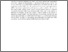# Design of fuzzy logic controller for AC motor based on field programmable gate array

## Citation

Obaid, Zeyad Assi and Sulaiman, Nasri and Hamidon, Mohd Nizar (2009) Design of fuzzy logic controller for AC motor based on field programmable gate array. In: 2009 IEEE Student Conference on Research and Development (SCOReD 2009), 16-18 Nov. 2009, UPM, Serdang, Selangor. (pp. 487-490).

## Abstract

This paper presents design of proportional-integral-derivative fuzzy logic controller (PIDFLC) to control the position in AC motor. Fuzzy logic controller has been designed using VHDL language for implementation in field programmable gate array (FPGA). Two version of the controller have been designed, the first one is 6-bits which uses 6-bits for each input/output variables (6FBC), while the second uses 8-bits for each input/output variables (8FBC). Second order mathematical model represents a position control in AC motor has been used in unity feedback control system with the proposed controller. Simulation environments have been built using non-synthesizable VHDL code for the purpose of simulation in ModelSim, and the same design is coded in Matlab for the purpose of simulation in Matlab (MSBC). The Mean differences between MSBC and 6FBC for Step response and control action are -0.0256 and -0.0009 respectively, and The Mean differences between MSBC and 8FBC for Step response and control action are -0.0030 and 0.0021 respectively, since the 8FBC is superior to 6FBC and its much close to MSBC.Preview
Text (Abstract)
Design of fuzzy logic controller for AC motor based on field programmable gate array.pdfView Item